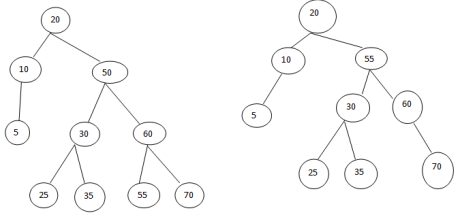# Binary search tree in Data Structures

21 September, 2018

Ratings

### Binary search tree

A binary search tree is a binary tree that may be empty A no-empty binary search tree in data structures satisfy the following properties :

• Every element has a key(or value), and no two elements have the same key; Therefore, all keys are distinct.
• The keys(if any) is the left subtree of the root are smaller than the key in the root.
• The keys(if any) in the right sub tree of the root are larger than the key in the root.
• The left and right sub trees at the root are also binary search trees.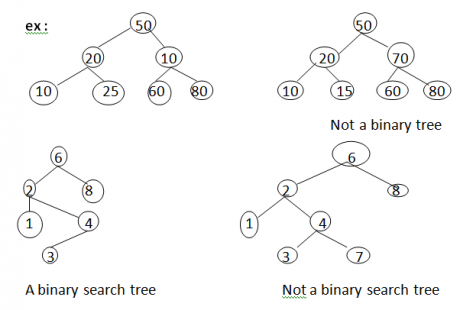Searching BST :

• If we are searching for 15, Then we are done
• If we are searching a key<15, Then we should search in the left sub tree
• If we are searching for a key > 15, then we should search in the right sub tree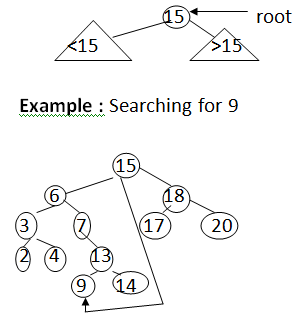### Search for 9:

1. compare 9: 15(The root), goto left sub tree;
2. Compare 9: 6,go to right sub tree.
3. compare 9: 7, go to right sub tee
4. compare 9: 9, found it

## Insertion operation

Algorithm:

1. perform search for value x
2. search will end at node y(if x not in tree)
3. if x<y, insert new leaf x as new left sub tree for y
4. if x>y, insert new leaf x as new right sub tree for y.

observations :

• 0(long(n)) operation for balanced tree
• Insertion may unbalance tree.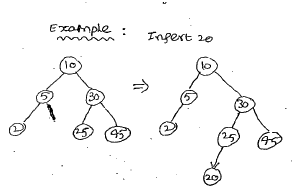10<20. right 30>20, left 25>20, left insert 20 on left Example : instruct a binary search tree with the following elements. 12,5,18,2,9,15,19,13,17 And traverse the binary search tree in zn order, preorder, post order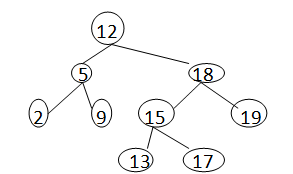Preorder : 12      529   1815131719 In order: 259       12     1315171819 Post order:295             131715191812

### Deletion operation :

For element removal we consider the three possibilities for the node P that contains the element to be removed.

• P is a leaf
• P has exactly one nonempty sub tree
• P has exactly two non empty sub tree

Case 1:          First cape is handled by discarding the  root, The root is set to null.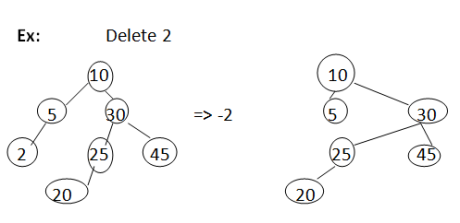Case 2: Next consider the case when the element to be removed is in ia node P that has only one non empty sub tree. IF P has no parent (i.e it is root), The root of its single sub tree becomes the new search tree root. IF p has a parent pp, then we change the pointer from PP so that it points to p’s only child. ex : Delete 5Case 3: Finally, to remove an element in a node that has two no empty sub trees, we replace this element with either the largest element in its left sub tree or the smallest element in its right sub tree. Following this replacement, the replacing element is removed from its original node Ex : Deleting 50